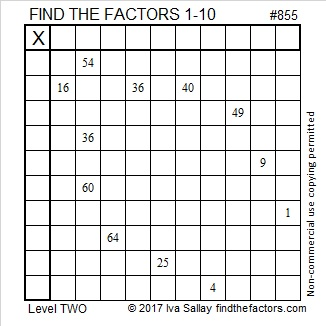# 855 A Bottle Full of Multiplication Facts

If you’ve always wanted to know the multiplication facts better, there is hope for you to do that in this bottle! Just write the numbers from 1 to 10 in the top row and also in the first column in an order that makes those factors and the given clues act like a multiplication table.Print the puzzles or type the solution on this excel file: 10-factors-853-863

855 is the hypotenuse of Pythagorean triple 513-684-855 which is (3, 4, 5) times 171.

From OEIS.org I learned that 855 can be expressed as sum of five consecutive squares (11² + 12² + 13² + 14² + 15² = 855) and the sum of two consecutive cubes (7³ + 8³ = 855). 855 is the smallest number that can make such a claim.

• 855 is a composite number.
• Prime factorization: 855 = 3 × 3 × 5 × 19, which can be written 855 = 3² × 5 × 19
• The exponents in the prime factorization are 2, 1, and 1. Adding one to each and multiplying we get (2 + 1)(1 + 1)(1 + 1) = 3 × 2 × 2 = 12. Therefore 855 has exactly 12 factors.
• Factors of 855: 1, 3, 5, 9, 15, 19, 45, 57, 95, 171, 285, 855
• Factor pairs: 855 = 1 × 855, 3 × 285, 5 × 171, 9 × 95, 15 × 57, or 19 × 45,
• Taking the factor pair with the largest square number factor, we get √855 = (√9)(√95) = 3√95 ≈ 29.240383